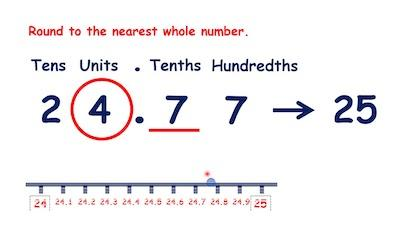# How To Round Decimals To The Nearest Whole Number

Rounding numbers doesn’t need to be hard. As long as you know the rules and methods that you need to use, you shouldn’t have any problems.

The reality is that there are many different rules and methods in what concerns rounding numbers. So, today, we decided to tell you a bit more about how you can easily round decimals to the nearest whole number.

Discover everything you need to know about rounding numbers.

## How To Round Decimals To The Nearest Whole Number

Ultimately, when you want or need to round decimals to the nearest whole number, you just need to keep in mind some basic rules.

The first thing that you need to do is to look at the first digit right after the decimal point. Then depending on the digit, you may need to leave the number as it is or you will need to round it up:

#1: When the digit is less than 5, which means that if the digit is 1, 2, 3, or 4, the number will stay the same.

#2: When the digit is 5 or greater, which means that if the digit is 5, 6, 7, 8, or 9, then you need to round up the number.

## How To Round Decimals To The Nearest Whole Number – Practical Examples

In our opinion, there’s nothing like checking some examples so that you know exactly how to proceed whenever you need to round decimals to the nearest whole number.

Example #1: Round 5.36 to the nearest whole number:

As we stated above, you will need to take a closer look at the digit that is on the right of the decimal point. In this case, it’s 3. Since 3 is less than 5, then you’ll need to round it to 5.

Looking to round a number to the nearest hundred?

Example #2: Round 32.74 to the nearest whole number:

As we stated above, you will need to take a closer look at the digit that is on the right of the decimal point. In this case, it’s 7. Since 7 is greater than 5, then you’ll need to round it up to 33.

Example #3: Round 9.63 to the nearest whole number:

As we stated above, you will need to take a closer look at the digit that is on the right of the decimal point. In this case, it’s 6. Since 6 is greater than 5, then you’ll need to round it up to 10.

Example #4: Round 78.537 to the nearest whole number:

As we stated above, you will need to take a closer look at the digit that is on the right of the decimal point. In this case, it’s 5. Since 5 is equal to 5, then you’ll need to round it up to 79.

## How To Round Decimals To Tenths, Hundredths, And Thousandths

When you want to round a decimal number, you will need to take a closer look at the digit after the one that you want to round to.

Use our rounding calculator to round your number to the nearest hundredth.

So, when you want to round a number to the nearest tenth, then you will need to take a closer look at the digit that is in the hundredths place. When you want to round a number to the nearest hundredth, you need to focus on the digit in the thousandths place.

Then, you just need to follow the rules that we previously mentioned:

#1: When the digit is less than 5, which means that if the digit is 1, 2, 3, or 4, the number will stay the same.

#2: When the digit is 5 or greater, which means that if the digit is 5, 6, 7, 8, or 9, then you need to round up the number.